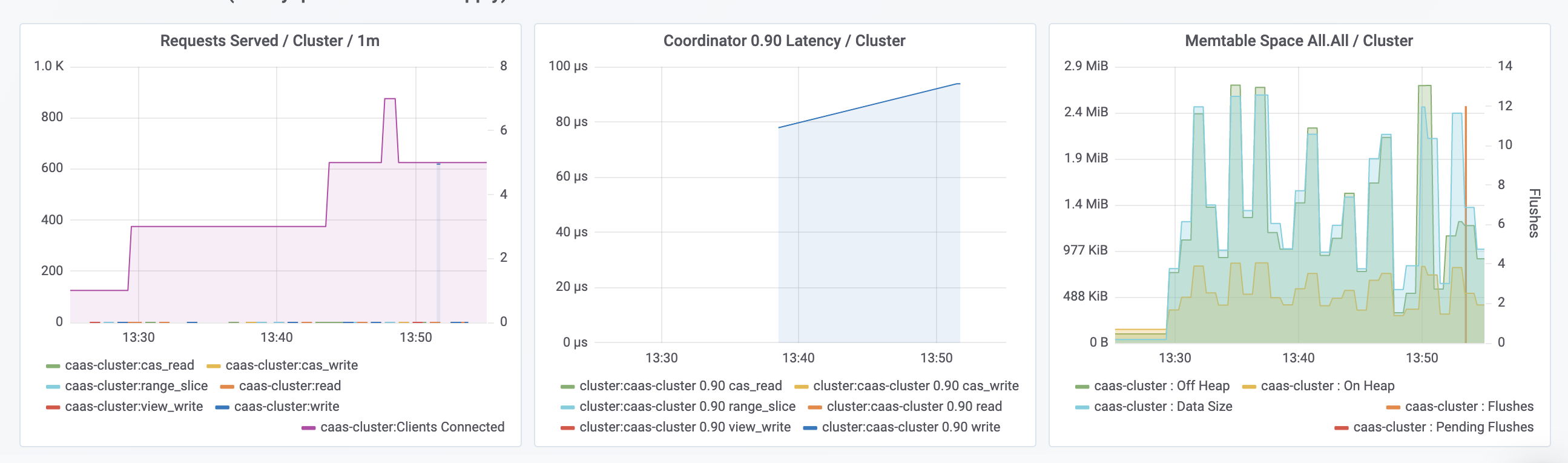Use NoSQLBench to test loading data to Astra with a demo cluster, keyspace, and user.

Prerequisites

1. Ensure you have a working NoSQLBench (nb or nb.jar). For more, see Getting NoSQLBench.

1. Make sure your Astra secure connect bundle is available to your nosqlbench instance. You can download the bundle from Astra and copy it to your /tmp directory.

Start Astra Benchmarking with NoSQLBench

nb cql-iot astra \
keyspace=demo \
secureconnectbundle=/tmp/secure-connect-demo.zip

or

java -jar nb.jar cql-iot astra \
keyspace=demo \
secureconnectbundle=/tmp/secure-connect-demo.zip

Sample Output

logging to logs/scenario_20201130_132903_455.log
cqliot_astra_schema: 100.00%/Stopped (details: min=0 cycle=1 max=1) (last report)
cqliot_astra_rampup: 0.37%/Stopped (details: min=0 cycle=36756 max=10000000)
cqliot_astra_rampup: 0.88%/Stopped (details: min=0 cycle=88159 max=10000000)
cqliot_astra_rampup: 1.40%/Stopped (details: min=0 cycle=139760 max=10000000)
cqliot_astra_rampup: 1.92%/Stopped (details: min=0 cycle=192445 max=10000000)
cqliot_astra_rampup: 2.44%/Stopped (details: min=0 cycle=243613 max=10000000)
cqliot_astra_rampup: 2.97%/Stopped (details: min=0 cycle=297122 max=10000000)
cqliot_astra_rampup: 3.51%/Stopped (details: min=0 cycle=350615 max=10000000)
cqliot_astra_rampup: 4.02%/Stopped (details: min=0 cycle=402398 max=10000000)
cqliot_astra_rampup: 4.45%/Stopped (details: min=0 cycle=444902 max=10000000)
cqliot_astra_rampup: 4.99%/Stopped (details: min=0 cycle=499381 max=10000000)
cqliot_astra_rampup: 5.53%/Stopped (details: min=0 cycle=552956 max=10000000)
cqliot_astra_rampup: 6.08%/Stopped (details: min=0 cycle=607688 max=10000000)
cqliot_astra_rampup: 6.56%/Stopped (details: min=0 cycle=656076 max=10000000)
cqliot_astra_rampup: 7.04%/Stopped (details: min=0 cycle=704018 max=10000000)
cqliot_astra_rampup: 7.55%/Stopped (details: min=0 cycle=754986 max=10000000)
cqliot_astra_rampup: 8.10%/Stopped (details: min=0 cycle=809898 max=10000000)
cqliot_astra_rampup: 8.89%/Stopped (details: min=0 cycle=888542 max=10000000)
cqliot_astra_rampup: 8.89%/Stopped (details: min=0 cycle=888542 max=10000000)
cqliot_astra_rampup: 9.19%/Stopped (details: min=0 cycle=919438 max=10000000)
cqliot_astra_rampup: 9.71%/Stopped (details: min=0 cycle=970703 max=10000000)
cqliot_astra_rampup: 10.25%/Stopped (details: min=0 cycle=1025088 max=10000000)
cqliot_astra_rampup: 10.71%/Stopped (details: min=0 cycle=1070983 max=10000000)
cqliot_astra_rampup: 11.20%/Stopped (details: min=0 cycle=1120478 max=10000000)
cqliot_astra_rampup: 11.68%/Stopped (details: min=0 cycle=1168035 max=10000000)
cqliot_astra_rampup: 12.19%/Stopped (details: min=0 cycle=1219462 max=10000000)

Check Out Health Tab